maths > mensuration-high

Perimeter & Area: Square, Rectangle, Triangle, Polygons

what you'll learn...

Overview

Finding perimeter and area of simple figures (squares, rectangle, triangle, polygon) are revised without much discussion.

perimeter

The word "perimeter" means: measure around a shape. Perimeter is from the Greek root words peri (around) and meter (measure).

Length is the distance-span between two points.

Perimeter :
Perimeter of a figure is the length of the line or curve forming the boundary of the figure.

Perimeter of square $=4×\phantom{\rule{1ex}{0ex}}\text{side}$$= 4 \times \textrm{s i \mathrm{de}}$

Perimeter of rectangle $=2×\phantom{\rule{1ex}{0ex}}\text{length + width}$$= 2 \times \textrm{\le n > h + w i \mathrm{dt} h}$

Perimeter of triangle $=\phantom{\rule{1ex}{0ex}}\text{sum of three sides}$$= \textrm{\sum o f t h r e e s i \mathrm{de} s}$

Perimeter of polygon $=\phantom{\rule{1ex}{0ex}}\text{sum of sides}$$= \textrm{\sum o f s i \mathrm{de} s}$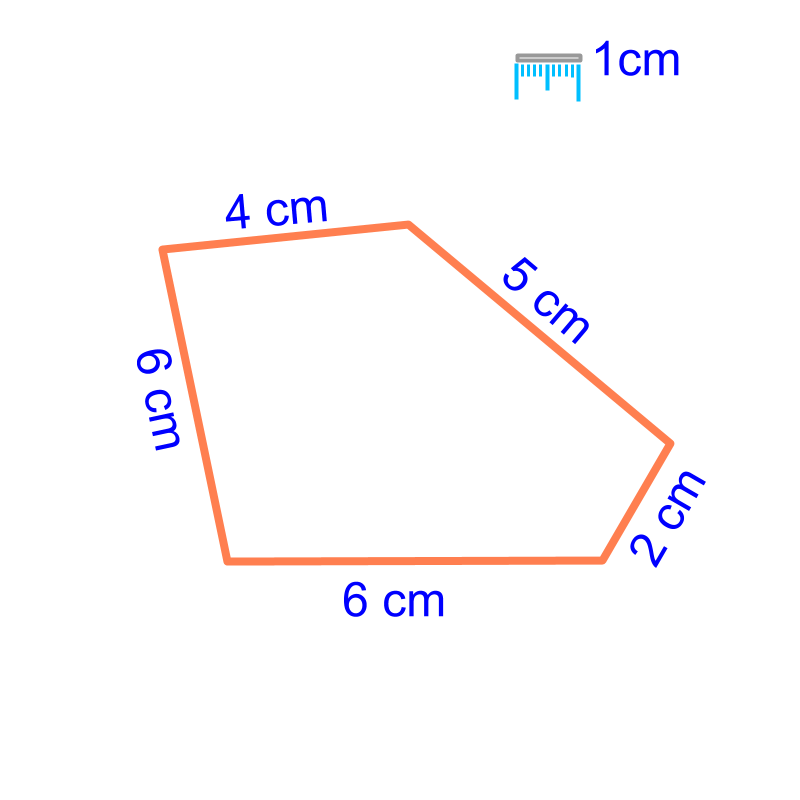area

Area of a figure is the "surface-span within a closed shape".

Area of a Square and a Rectangle :
Area of square $={\phantom{\rule{1ex}{0ex}}\text{side}}^{2}$$= {\textrm{s i \mathrm{de}}}^{2}$

Area of rectangle $=\phantom{\rule{1ex}{0ex}}\text{length}\phantom{\rule{1ex}{0ex}}×\phantom{\rule{1ex}{0ex}}\text{width}$$= \textrm{\le n > h} \times \textrm{w i \mathrm{dt} h}$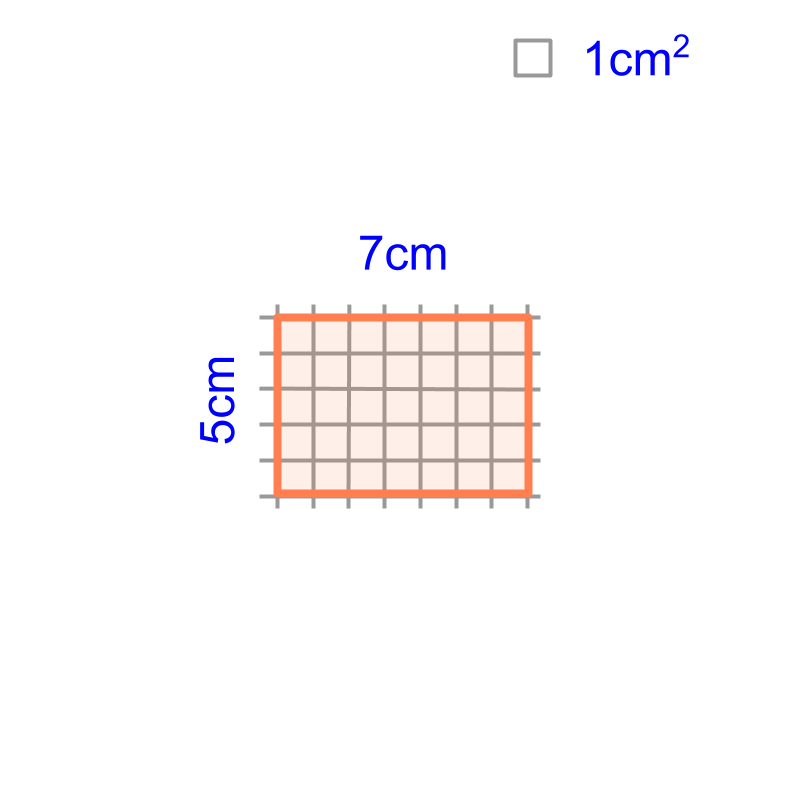Area of a Triangle:
$\frac{1}{2}×\phantom{\rule{1ex}{0ex}}\text{base}\phantom{\rule{1ex}{0ex}}×\left(height\right)$$\frac{1}{2} \times \textrm{b a s e} \times \left(h e i g h t\right)$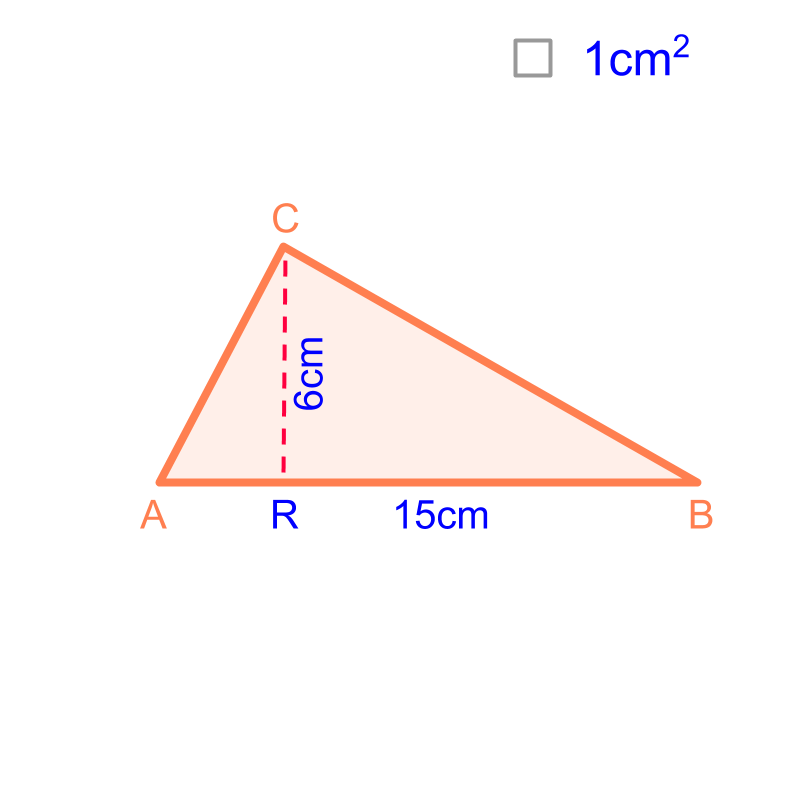Area of a Polygon : Consider a polygon to be combination of known geometrical forms, mostly triangles.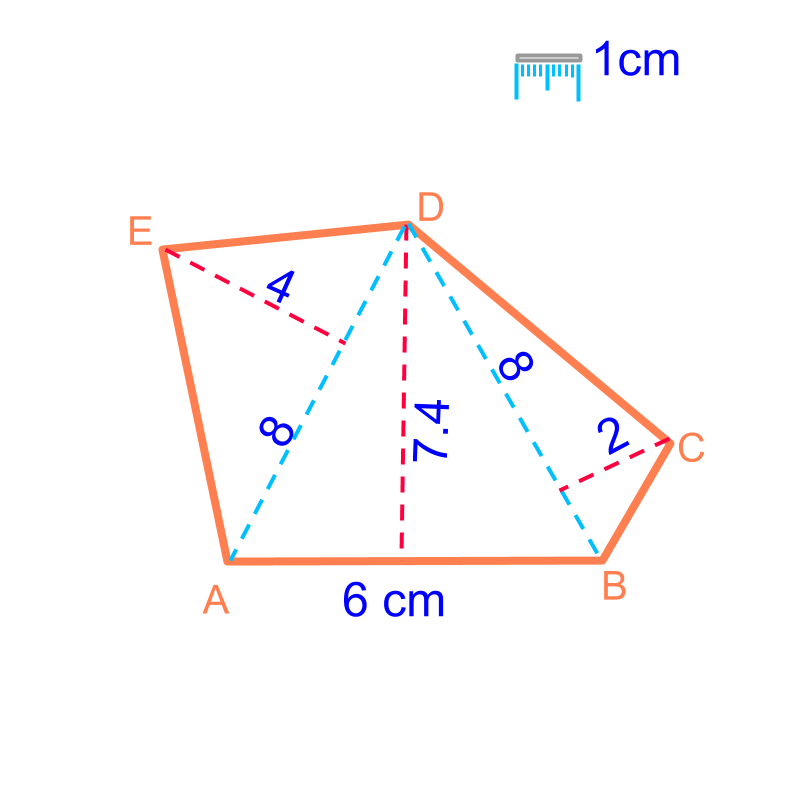The geometrical forms and the formula for area are:

Area of a triangle $=\frac{1}{2}×\phantom{\rule{1ex}{0ex}}\text{base}\phantom{\rule{1ex}{0ex}}×\phantom{\rule{1ex}{0ex}}\text{height}$$= \frac{1}{2} \times \textrm{b a s e} \times \textrm{h e i g h t}$

Area of a trapezium $=\frac{1}{2}×\phantom{\rule{1ex}{0ex}}\text{sum of bases}\phantom{\rule{1ex}{0ex}}×\phantom{\rule{1ex}{0ex}}\text{height}$$= \frac{1}{2} \times \textrm{\sum o f b a s e s} \times \textrm{h e i g h t}$

Area of a parallelogram $=\phantom{\rule{1ex}{0ex}}\text{base}\phantom{\rule{1ex}{0ex}}×\phantom{\rule{1ex}{0ex}}\text{height}$$= \textrm{b a s e} \times \textrm{h e i g h t}$

Area of a kite $=\frac{1}{2}×\phantom{\rule{1ex}{0ex}}\text{major-diagonal}\phantom{\rule{1ex}{0ex}}×\phantom{\rule{1ex}{0ex}}\text{minor-diagonal}$$= \frac{1}{2} \times \textrm{m a j \mathmr{and} - \mathrm{di} a g o n a l} \times \textrm{\min \mathmr{and} - \mathrm{di} a g o n a l}$

Area of Some Quadrilaterals : Consider the polygon shapes as combination of triangles and find sum of area of the triangles.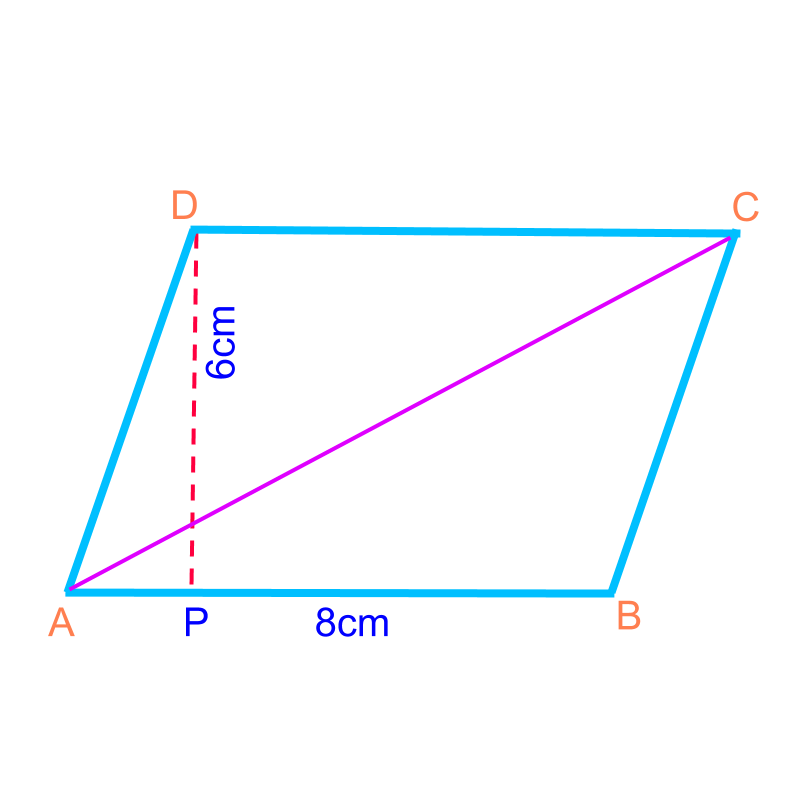$\phantom{\rule{1ex}{0ex}}\text{area of a parallelogram}\phantom{\rule{1ex}{0ex}}$$\textrm{a r e a o f a p a r a l \le \log r a m}$$=\phantom{\rule{1ex}{0ex}}\text{base}\phantom{\rule{1ex}{0ex}}×\phantom{\rule{1ex}{0ex}}\text{height}$$= \textrm{b a s e} \times \textrm{h e i g h t}$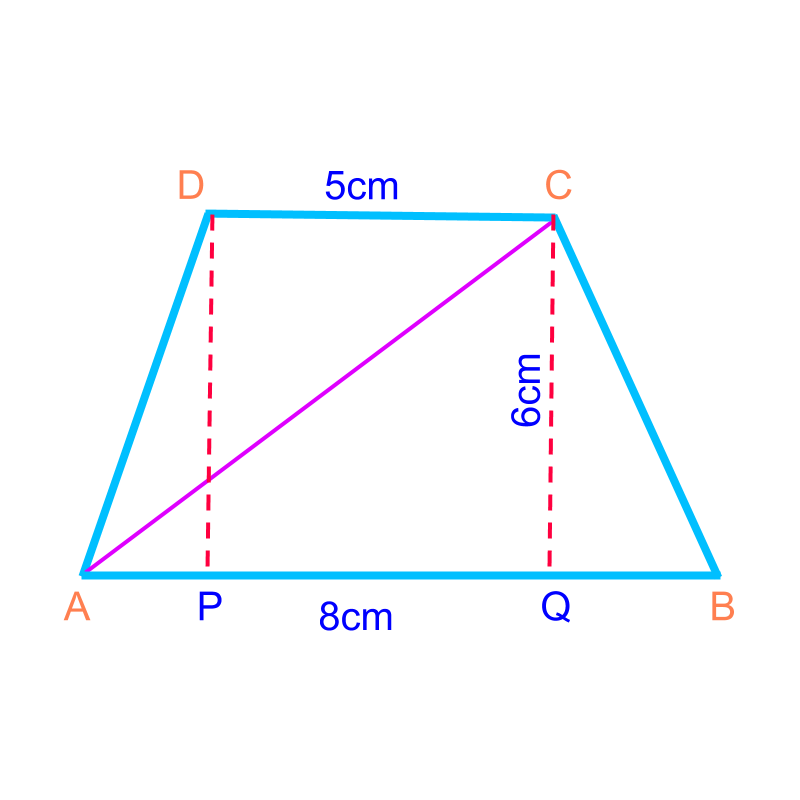$\phantom{\rule{1ex}{0ex}}\text{area of a trapezium}\phantom{\rule{1ex}{0ex}}$$\textrm{a r e a o f a t r a p e z i u m}$$=\frac{1}{2}×\phantom{\rule{1ex}{0ex}}\text{sum of bases}\phantom{\rule{1ex}{0ex}}×\phantom{\rule{1ex}{0ex}}\text{height}$$= \frac{1}{2} \times \textrm{\sum o f b a s e s} \times \textrm{h e i g h t}$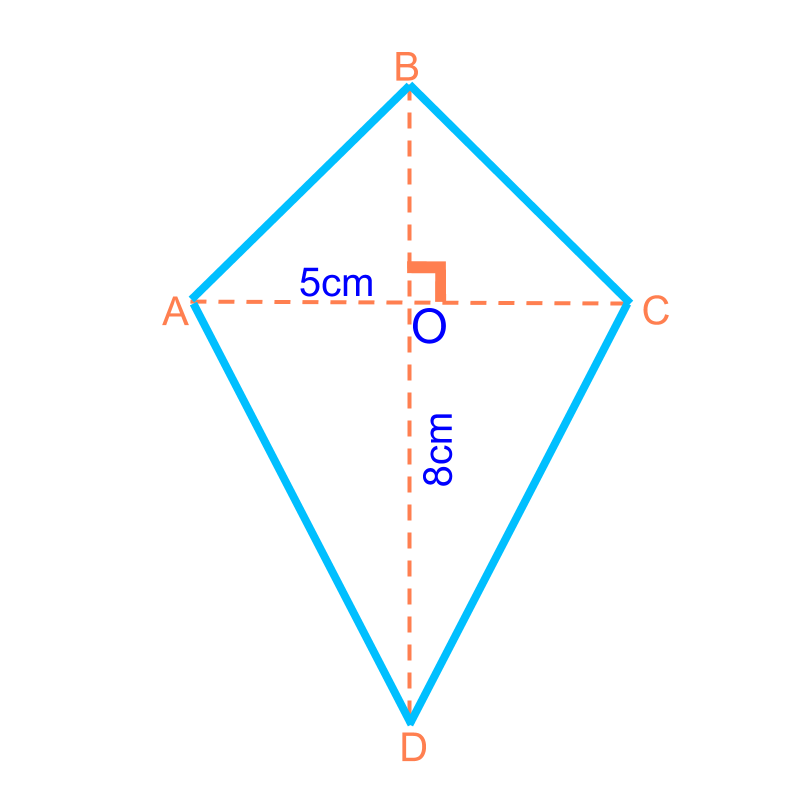$\phantom{\rule{1ex}{0ex}}\text{area of a kite}\phantom{\rule{1ex}{0ex}}$$\textrm{a r e a o f a k i t e}$$=\frac{1}{2}×\phantom{\rule{1ex}{0ex}}\text{product of the diagonals}$$= \frac{1}{2} \times \textrm{\prod u c t o f t h e \mathrm{di} a g o n a l s}$

summary

This is covered in Mesuration-Basics in the following pages

→   Perimeter of Polygons

→   Area of Square & rectangle

→   Area of Triangle

→   Area of Polygons

→   Perimeter & Area of Quadrilaterals

Outline